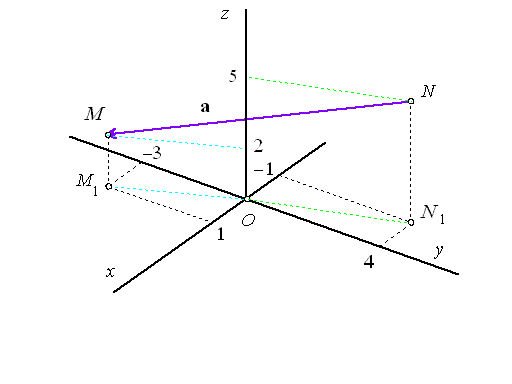Example 8. From homogeneoues coordinates find Cartesian coordinates of real point $M=\left(2,-6,4,2\right)$ and of vector $\mathbf{a}=MN,\phantom{\rule{2mm}{2mm}}N=\left(-2,8,10,2\right).$

Solution. Cartesian coordinates of the real point M form a triple of real numbers

 $M=\left(\frac{{a}_{1}}{{a}_{0}},\frac{{a}_{2}}{{a}_{0}},\frac{{a}_{3}}{{a}_{0}}\right)=\left(\frac{2}{2},\phantom{\rule{2mm}{2mm}}\frac{-6}{2},\phantom{\rule{2mm}{2mm}}\frac{4}{2}\right)=\left(1,-3,2\right)$

where ${a}_{0}=2$ is the last homogeneous coordinate.

Cartesian coordinates of the vector $\mathbf{a}=MN$ can be derived from the Cartesian coordinates of the endpoints, while

 $N=\left(\frac{-2}{2},\frac{8}{2},\frac{10}{2}\right)=\left(-1,4,5\right)$

and coordinates of the vetor are

 $\mathbf{a}=\stackrel{\to }{MN}=N-M=\left(-1,4,5\right)-\left(1,-3,2\right)=\left(-2,7,3\right)$Figure 1: Cartesian coordinates